# How to Look Up the Lowest Value in A List by VLOOKUP/INDEX/MATCH Functions in Excel

VLOOKUP function is very useful in our daily work and we can use it to look up match value in a range, then get proper returned value (the returned value may be just adjacent to the match value). Sometimes we only want to look up the lowest value among all matched values in the list and get its adjacent value, how can we do? This tutorial will help you to look up the lowest value in a list by VLOOKUP function. However, except VLOOKUP function, we can also use INDEX/MATCH functions together in some situations to look up the lowest value as well. Please see details below.

Precondition:

Prepare a table consists of name, score and range columns. Now we want to get the ‘Range’ of the lowest score.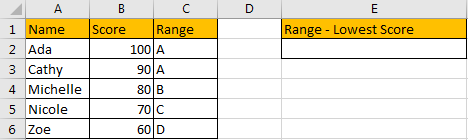## Method 1: Look Up the Lowest Value by VLOOKUP Function

Step 1: In E2, enter the formula =VLOOKUP(MIN(B2:B6),B2:C6,2,FALSE). As we want to find the ‘Range’ of the lowest score, we need to look up the lowest score among all score first, so we enter MIN(B2:B6) as lookup_value.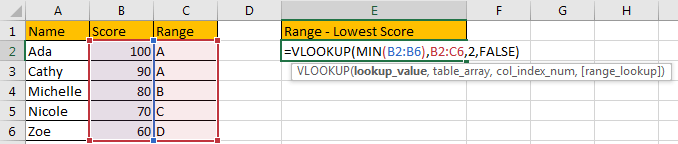Step 2: Click Enter to get returned value in C column.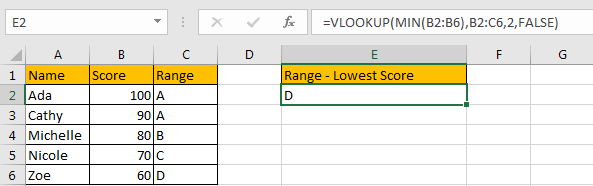Comment:

1.If there are two duplicate lowest values, VLOOKUP function will look up the first match value and return its adjacent value.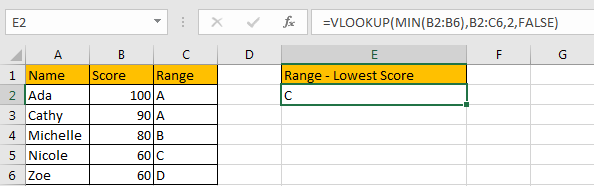2.This method only works well when returned value is listed in the right column.

## Method 2: Look Up the Lowest Value by INDEX/MATCH Functions

In above example, if we want to know the name of the lowest score, VLOOKUP function doesn’t work. So we use INDEX and MATCH functions combination to look up the lowest value here.

Step 1: In B2, enter the formula =INDEX(A2:A6,MATCH(MIN(B2:B6),B2:B6,0)). MATCH function returns the location of cell, in this example it returns the lowest value’s row number. Then we can use INDEX function to get proper Name from A2:A6 refer to row number.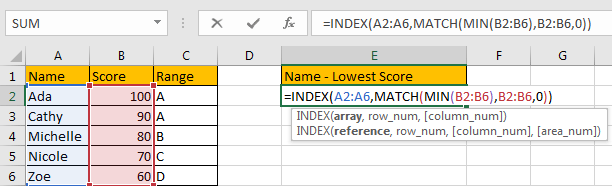Step 2: Click Enter to get returned value.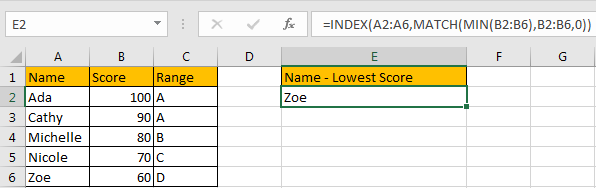Comment:

1.If there are two duplicate lowest values, INDEX/MATCH functions will look up the first match value and return its adjacent value.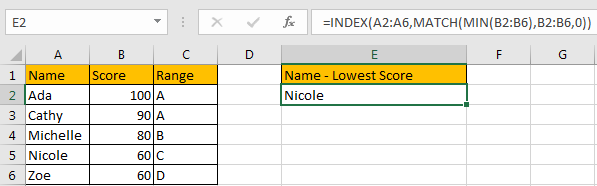2.This method works well for that returned value lists on the both sides of match value.

### Related Functions

• Excel INDEX function
The Excel INDEX function returns a value from a table based on the index (row number and column number)The INDEX function is a build-in function in Microsoft Excel and it is categorized as a Lookup and Reference Function.The syntax of the INDEX function is as below:= INDEX (array, row_num,[column_num])…
• Excel MAX function
The Excel MAX function returns the largest numeric value from the numbers that you provided. Or returns the largest value in the array.= MAX(num1,[num2,…numn])…
• Excel MIN function
The Excel MIN function returns the smallest numeric value from the numbers that you provided. Or returns the smallest value in the array.The MIN function is a build-in function in Microsoft Excel and it is categorized as a Statistical Function.The syntax of the MIN function is as below:= MIN(num1,[num2,…numn])….
• Excel VLOOKUP function
The Excel VLOOKUP function lookup a value in the first column of the table and return the value in the same row based on index_num position.The syntax of the VLOOKUP function is as below:= VLOOKUP (lookup_value, table_array, column_index_num,[range_lookup])….
• Excel MATCH  function
The Excel MATCH function search a value in an array and returns the position of that item.The MATCH function is a build-in function in Microsoft Excel and it is categorized as a Lookup and Reference Function.The syntax of the MATCH function is as below:= MATCH  (lookup_value, lookup_array, [match_type])….
Related Posts

Build Hyperlink With VLOOKUP in Excel

You might have come across a task in which you were assigned to build hyperlinks, which seems very easy, and if you are new to excel or don't have enough experience with it, then you might wonder about doing this ...

Calculate Total Cost with Excel VLOOKUP Function

In today's article we will show you how to calculate the total cost for a given weight using the Excel VLOOKUP function. This function will help us to find the appropriate unit price for that weight and then we can ...

Basic Price Discount Calculation with Excel VLOOKUP Function

We often encounter product discounts in our shopping. Depending on the level of spending, the mall will offer different percentages of discounts. Usually, the more you spend, the bigger the discount, while the less you spend, the smaller the discount. ...

Calculate Grades With VLookup in Excel

Why Should You Calculate Grades With VLookup Excel? If you're looking for a simple way to find out a student's grades, VLOOKUP Excel can do the trick. This function uses a lookup table to find the values and sort them ...

Convert State Names To Abbreviations

Assume that you got a task to convert the full state's name into the abbreviations in MS Excel, and for doing this task, you might choose to do it manually, which is an acceptable way only if you don't have ...

Find and Replace Multiple Values

This post will guide you how to find and replace multiple values at once with VBA macro or using formula  in Excel. How do I make multiple find and replace in Excel. Suppose that you have a few cells containing ...

Excel XLOOKUP Function

Excel XLOOKUP Function was added into Excel as a beta feature in August 2019 and is now accessible exclusively in Microsoft 365. (as of July 2021). However, if you fall into this category and often deal with big sets of ...

VLOOKUP Formula | Faster Trick with 2 VLOOKUPS

This post will guide you how to use 2 VLOOKUPS function to looking up data entries from a given range of cells in Microsoft Excel. VLOOKUP with 2 lookups can be faster than a single VLOOKUP in certain scenarios. The ...

VBA Macro For VLOOKUP From Another Sheet

In the previous post, you should know that how to fix or remove the #N/A error when using VLOOKUP formula to lookup value from another sheet. And this post will show you how to use VBA code to vlookup data ...

VLOOKUP From Another Sheet Not Working

In the previous post, you should know that how to fix or remove the #N/A error when using VLOOKUP formula to lookup value from another sheet. And this post will show you reasons why your VLOOKUP formula is not working ...

Sidebar